# Mirror Images Quiz II, Non Verbal Reasoning

## 25 Questions MCQ Test Non Verbal Reasoning | Mirror Images Quiz II, Non Verbal Reasoning

Description
Attempt Mirror Images Quiz II, Non Verbal Reasoning | 25 questions in 50 minutes | Mock test for LR preparation | Free important questions MCQ to study Non Verbal Reasoning for LR Exam | Download free PDF with solutions
QUESTION: 1

Solution:
QUESTION: 2

Solution:
QUESTION: 3

### Directions to Solve In each of the following questions, choose the correct mirror images of the given image of the Fig.(X) from amongst the four alternatives (1), (2), (3) and (4) given along with it. Question - Choose the correct mirror image of the given figure (X) from amongst the four alternatives.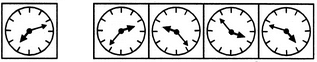(X)                 (1)          (2)         (3)         (4)

Solution:
QUESTION: 4

Directions to Solve

In each of the following questions, choose the correct mirror images of the given image of the Fig.(X) from amongst the four alternatives (1), (2), (3) and (4) given along with it.

Question -

Choose the correct mirror image of the given figure (X) from amongst the four alternatives.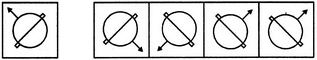(X)                 (1)          (2)         (3)         (4)

Solution:
QUESTION: 5

In each of the following questions, choose the correct mirror images of the given image of the Fig.(X) from amongst the four alternatives (1), (2), (3) and (4) given along with it.

Question -

Choose the correct mirror image of the given figure (X) from amongst the four alternatives.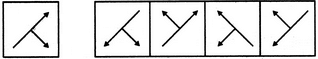(X)                 (1)          (2)         (3)         (4)
Solution:
QUESTION: 6

In each of the following questions, choose the correct mirror images of the given image of the Fig.(X) from amongst the four alternatives (1), (2), (3) and (4) given along with it.

Question -

Choose the correct mirror image of the given figure (X) from amongst the four alternatives.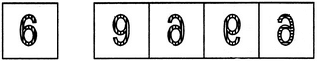(X)                 (1)          (2)         (3)         (4)
Solution:
QUESTION: 7

In each of the following questions, choose the correct mirror images of the given image of the Fig.(X) from amongst the four alternatives (1), (2), (3) and (4) given along with it.

Question -

Choose the correct mirror image of the given figure (X) from amongst the four alternatives.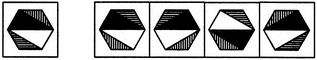(X)                 (1)          (2)         (3)         (4)
Solution:
QUESTION: 8

In each of the following questions, choose the correct mirror images of the given image of the Fig.(X) from amongst the four alternatives (1), (2), (3) and (4) given along with it.

Question -

Choose the correct mirror image of the given figure (X) from amongst the four alternatives.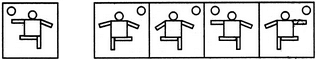(X)                 (1)          (2)         (3)         (4)
Solution:
QUESTION: 9

In each of the following questions, choose the correct mirror images of the given image of the Fig.(X) from amongst the four alternatives (1), (2), (3) and (4) given along with it.

Question -

Choose the correct mirror image of the given figure (X) from amongst the four alternatives.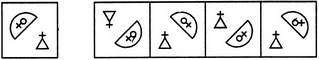(X)                 (1)          (2)         (3)         (4)
Solution:
QUESTION: 10

In each of the following questions, choose the correct mirror images of the given image of the Fig.(X) from amongst the four alternatives (1), (2), (3) and (4) given along with it.

Question -

Choose the correct mirror image of the given figure (X) from amongst the four alternatives.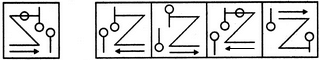(X)                 (1)          (2)         (3)         (4)
Solution:
QUESTION: 11

In each of the following questions, choose the correct mirror images of the given image of the Fig.(X) from amongst the four alternatives (1), (2), (3) and (4) given along with it.

Question -

Choose the correct mirror image of the given figure (X) from amongst the four alternatives.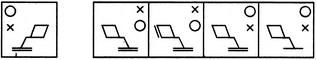(X)                 (1)          (2)         (3)         (4)
Solution:
QUESTION: 12

In each of the following questions, choose the correct mirror images of the given image of the Fig.(X) from amongst the four alternatives (1), (2), (3) and (4) given along with it.

Question -

Choose the correct mirror image of the given figure (X) from amongst the four alternatives.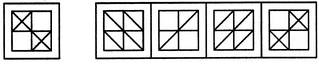(X)                 (1)          (2)         (3)         (4)
Solution:
QUESTION: 13

In each of the following questions, choose the correct mirror images of the given image of the Fig.(X) from amongst the four alternatives (1), (2), (3) and (4) given along with it.

Question -

Choose the correct mirror image of the given figure (X) from amongst the four alternatives.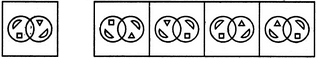(X)                 (1)          (2)         (3)         (4)
Solution:
QUESTION: 14

In each of the following questions, choose the correct mirror images of the given image of the Fig.(X) from amongst the four alternatives (1), (2), (3) and (4) given along with it.

Question -

Choose the correct mirror image of the given figure (X) from amongst the four alternatives.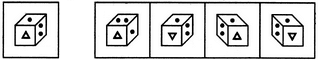(X)                 (1)          (2)         (3)         (4)
Solution: Flip the given image Horizontally to get mirror image.
QUESTION: 15

In each of the following questions, choose the correct mirror images of the given image of the Fig.(X) from amongst the four alternatives (1), (2), (3) and (4) given along with it.

Question -

Choose the correct mirror image of the given figure (X) from amongst the four alternatives.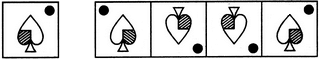(X)                 (1)          (2)         (3)         (4)
Solution:
QUESTION: 16

In each of the following questions, choose the correct mirror images of the given image of the Fig.(X) from amongst the four alternatives (1), (2), (3) and (4) given along with it.

Question -

Choose the correct mirror image of the given figure (X) from amongst the four alternatives.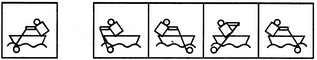(X)                 (1)          (2)         (3)         (4)
Solution:
QUESTION: 17

In each of the following questions, choose the correct mirror images of the given image of the Fig.(X) from amongst the four alternatives (1), (2), (3) and (4) given along with it.

Question -

Choose the correct mirror image of the given figure (X) from amongst the four alternatives.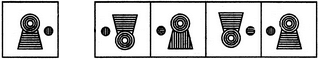(X)                 (1)          (2)         (3)         (4)
Solution:
QUESTION: 18

In each of the following questions, choose the correct mirror images of the given image of the Fig.(X) from amongst the four alternatives (1), (2), (3) and (4) given along with it.

Question -

Choose the correct mirror image of the given figure (X) from amongst the four alternatives.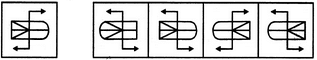(X)                 (1)          (2)         (3)         (4)
Solution:
QUESTION: 19

In each of the following questions, choose the correct mirror images of the given image of the Fig.(X) from amongst the four alternatives (1), (2), (3) and (4) given along with it.

Question -

Choose the correct mirror image of the given figure (X) from amongst the four alternatives.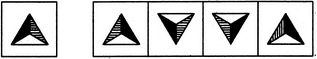(X)                 (1)          (2)         (3)         (4)
Solution:
QUESTION: 20

In each of the following questions, choose the correct mirror images of the given image of the Fig.(X) from amongst the four alternatives (1), (2), (3) and (4) given along with it.

Question -

Choose the correct mirror image of the given figure (X) from amongst the four alternatives.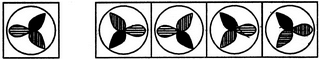(X)                 (1)          (2)         (3)         (4)
Solution:
QUESTION: 21

In each of the following questions, choose the correct mirror images of the given image of the Fig.(X) from amongst the four alternatives (1), (2), (3) and (4) given along with it.

Question -

Choose the correct mirror image of the given figure (X) from amongst the four alternatives.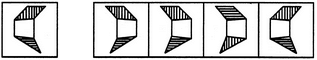(X)                 (1)          (2)         (3)         (4)
Solution:
QUESTION: 22

In each of the following questions, choose the correct mirror images of the given image of the Fig.(X) from amongst the four alternatives (1), (2), (3) and (4) given along with it.

Question -

Choose the correct mirror image of the given figure (X) from amongst the four alternatives.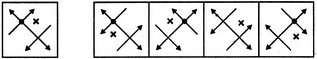(X)                 (1)          (2)         (3)         (4)
Solution:
QUESTION: 23

In each of the following questions, choose the correct mirror images of the given image of the Fig.(X) from amongst the four alternatives (1), (2), (3) and (4) given along with it.

Question -

Choose the correct mirror image of the given figure (X) from amongst the four alternatives.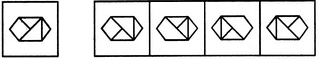(X)                 (1)          (2)         (3)         (4)
Solution:
QUESTION: 24

In each of the following questions, choose the correct mirror images of the given image of the Fig.(X) from amongst the four alternatives (1), (2), (3) and (4) given along with it.

Question -

Choose the correct mirror image of the given figure (X) from amongst the four alternatives.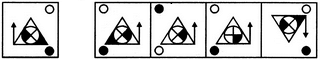(X)                 (1)          (2)         (3)         (4)
Solution:
QUESTION: 25

In each of the following questions, choose the correct mirror images of the given image of the Fig.(X) from amongst the four alternatives (1), (2), (3) and (4) given along with it.

Question -

Choose the correct mirror image of the given figure (X) from amongst the four alternatives.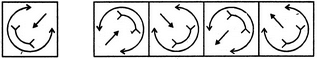(X)                 (1)          (2)         (3)         (4)
Solution:Use Code STAYHOME200 and get INR 200 additional OFF Use Coupon Code